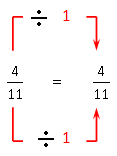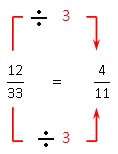PRE-ALGEBRA
ALGEBRA 1
GEOMETRY
ALGEBRA 2
TRIGONOMETRY
PRE-CALCULUS
CALCULUSCalifornia Algebra 1, 2008PAGE: 108 SET: Exercises PROBLEM: 1
Please look in your text book for this problem StatementAn equation stating that two ratios are equal is called a proportion.

Method 1:

Ratio of the first fractionRatio of the second fractionCheck the ratios of fractions.

The ratios are equal. So, the ratios form a proportion.Method 2:

Write the equationAccording to Means-extremes Property of proportion: If, then.(Cross products)(Multiply:)

The cross products are equal. So, the ratios form a proportion.Yes, the ratios form a proportion..TESTIMONIALS
 "I want to tell you that our students did well on the math exam and showed a marked improvement that, in my estimation, reflected the professional development the faculty received from you. THANK YOU!!!" June Barnett "Your site is amazing! It helped me get through Algebra." Charles "My daughter uses it to supplement her Algebra 1 school work. She finds it very helpful." Dan Pease
 Tweets by @mathskeydotcom

QUESTIONS? LET US HELP.
mathskey.com is not affiliated with any Publisher, Book cover, Title, Author names appear for reference only